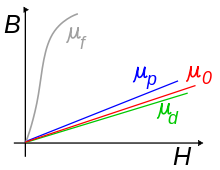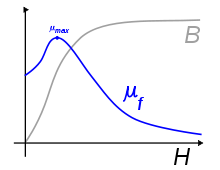# Permeability (electromagnetism)

Permeability (electromagnetism)
Magnetic Circuits

Conventional Magnetic Circuits
• Magnetomotive force$\mathcal F$
• Magnetic flux Φ
• Magnetic reluctance$\mathcal R$

Phasor Magnetic Circuits

Related Concepts
• Magnetic permeability μ

Gyrator-capacitor model variables
This box:Simplified comparison of permeabilities for: ferromagnetsf), paramagnetsp), free space(μ0) and diamagnetsd)

In electromagnetism, permeability is the measure of the ability of a material to support the formation of a magnetic field within itself. In other words, it is the degree of magnetization that a material obtains in response to an applied magnetic field. Magnetic permeability is typically represented by the Greek letter μ. The term was coined in September, 1885 by Oliver Heaviside. The reciprocal of magnetic permeability is magnetic reluctivity.

We can simplify it by saying, the more conductive a material is to a magnetic field, the higher its permeability.

In SI units, permeability is measured in the henry per metre (H·m−1), or newton per ampere squared (N·A−2). The permeability constant (μ0), also known as the magnetic constant or the permeability of free space, is a measure of the amount of resistance encountered when forming a magnetic field in a classical vacuum. The magnetic constant has the exact (defined) value µ0 = 4π×10−7 ≈ 1.2566370614…×10−6 H·m−1 or N·A−2).

## Explanation

In electromagnetism, the auxiliary magnetic field H represents how a magnetic field B influences the organization of magnetic dipoles in a given medium, including dipole migration and magnetic dipole reorientation. Its relation to permeability is$\mathbf{B}=\mu \mathbf{H}$

where the permeability μ is a scalar if the medium is isotropic or a second rank tensor for an anisotropic medium.

In general, permeability is not a constant, as it can vary with the position in the medium, the frequency of the field applied, humidity, temperature, and other parameters. In a nonlinear medium, the permeability can depend on the strength of the magnetic field. Permeability as a function of frequency can take on real or complex values. In ferromagnetic materials, the relationship between B and H exhibits both non-linearity and hysteresis: B is not a single-valued function of H, but depends also on the history of the material. For these materials it is sometimes useful to consider the incremental permeability defined as$\Delta\mathbf{B}=\mu_{\Delta} \Delta\mathbf{H}$.

This definition is useful in local linearizations of non-linear material behavior, for example in a Newton-Raphson iterative solution scheme that computes the changing saturation of a magnetic circuit.

Permeability is the inductance per unit length. In SI units, permeability is measured in henries per metre (H·m−1 = J/(A2·m) = N A−2). The auxiliary magnetic field H has dimensions current per unit length and is measured in units of amperes per metre (A m−1). The product μH thus has dimensions inductance times current per unit area (H·A/m2). But inductance is magnetic flux per unit current, so the product has dimensions magnetic flux per unit area. This is just the magnetic field B, which is measured in webers (volt-seconds) per square-metre (V·s/m2), or teslas (T).

B is related to the Lorentz force on a moving charge q:$\mathbf{F} = q (\mathbf{E} + \mathbf{v} \times \mathbf{B})$.

The charge q is given in coulombs (C), the velocity v in m/s, so that the force F is in newtons (N):$q \mathbf{v} \times \mathbf{B} = \mbox{C} \cdot \dfrac{\mbox{m}}{\mbox{s}} \cdot \dfrac{\mbox{V} \cdot \mbox{s}}{\mbox{m}^2} = \dfrac{\mbox{C} \cdot (\mbox{J / C})}{\mbox{m}} = \dfrac{\mbox{J}}{\mbox{m}} = \mbox{N}$

H is related to the magnetic dipole density. A magnetic dipole is a closed circulation of electric current. The dipole moment has dimensions current times area, units ampere square-metre (A·m2), and magnitude equal to the current around the loop times the area of the loop. The H field at a distance from a dipole has magnitude proportional to the dipole moment divided by distance cubed, which has dimensions current per unit length.

## Relative permeability

Relative permeability, sometimes denoted by the symbol μr, is the ratio of the permeability of a specific medium to the permeability of free space given by the magnetic constant$\mu_0 = 4 \pi \times 10^{-7} \frac{N}{A^2}$:$\mu_{r} = \frac{\mu}{\mu_{0}}$.

In terms of relative permeability, the magnetic susceptibility is:

χm = μr − 1.

χm, a dimensionless quantity, is sometimes called volumetric or bulk susceptibility, to distinguish it from χp (magnetic mass or specific susceptibility) and χM (molar or molar mass susceptibility).

## Diamagnetism

Diamagnetism is the property of an object which causes it to create a magnetic field in opposition of an externally applied magnetic field, thus causing a repulsive effect. Specifically, an external magnetic field alters the orbital velocity of electrons around their nuclei, thus changing the magnetic dipole moment in the direction opposing the external field. Diamagnets are materials with a magnetic permeability less than μ0 (a relative permeability less than 1).

Consequently, diamagnetism is a form of magnetism that a substance exhibits only in the presence of an externally applied magnetic field. It is generally a quite weak effect in most materials, although superconductors exhibit a strong effect.

## Paramagnetism

Paramagnetism is a form of magnetism which occurs only in the presence of an externally applied magnetic field. Paramagnetic materials are attracted to magnetic fields, hence have a relative magnetic permeability greater than one (or, equivalently, a positive magnetic susceptibility). The magnetic moment induced by the applied field is linear in the field strength and rather weak. It typically requires a sensitive analytical balance to detect the effect. Unlike ferromagnets, paramagnets do not retain any magnetization in the absence of an externally applied magnetic field, because thermal motion causes the spins to become randomly oriented without it. Thus the total magnetization will drop to zero when the applied field is removed. Even in the presence of the field there is only a small induced magnetization because only a small fraction of the spins will be oriented by the field. This fraction is proportional to the field strength and this explains the linear dependency. The attraction experienced by ferromagnets is non-linear and much stronger, so that it is easily observed, for instance, in magnets on one's refrigerator.

## Values for some common materials

The following table should be used with caution as the permeability of ferromagnetic materials varies greatly with field strength. For example 4% Si steel has an initial relative permeability (at or near 0T) of 2,000 and a maximum of 35,000 and, indeed, the relative permeability of any material at a sufficiently high field strength tends to 1.

Magnetic susceptibility and permeability data for selected materials
Medium Susceptibility χm
(volumetric SI)
Permeability μ [H/m] Relative Permeability μ/μ0 Magnetic field Frequency max.
Metglas 1.25×10−1 1,000,000 at 0.5 T 100kHz
Nanoperm 10×10−2 80,000 at 0.5 T 10kHz
Mu-metal 2.5×10−2 20,000 at 0.002 T
Mu-metal 50,000
Permalloy 1.0×10−2 8,000 at 0.002 T
Electrical steel 5.0×10−3 4,000 at 0.002 T
Ferrite (nickel zinc) 2.0×10−58.0×10−4 16–640 100 kHz ~ 1 MHz
Ferrite (manganese zinc) >8.0×10−4 640 (or more) 100 kHz ~ 1 MHz
Steel 8.75×10−4 100 at 0.002 T
Nickel 1.25×10−4 100 – 600 at 0.002 T
Neodymium magnet 1.05
Platinum 1.2569701×10−6 1.000265
Aluminum 2.22×10−5 1.2566650×10−6 1.000022
Wood 1.00000043
Air 1.00000037 
Concrete 1
Vacuum 0 1.2566371×10−60) 1
Hydrogen −2.2×10−9 1.2566371×10−6 1.0000000
Teflon 1.2567×10−6 1.0000
Sapphire −2.1×10−7 1.2566368×10−6 0.99999976
Copper −6.4×10−6
or −9.2×10−6
1.2566290×10−6 0.999994
Water −8.0×10−6 1.2566270×10−6 0.999992
Bismuth −1.66×10−4 0.999834
Superconductors −1 0 0Magnetisation curve for ferromagnets (and ferrimagnets) and corresponding permeability

A good magnetic core material must have high permeability.[citation needed]

For magnetic levitation a relative permeability below 1 is needed (corresponding to a negative susceptibility).

Permeability varies with magnetic field. Values shown above are approximate and valid only at the magnetic fields shown. Moreover, they are given for a zero frequency; in practice, the permeability is generally a function of the frequency. When frequency is considered the permeability can be complex, corresponding to the in phase and out of phase response.

Note that the magnetic constant μ0 has an exact value in SI units (that is, there is no uncertainty in its value), because the definition of the ampere fixes its value to 4π × 10−7 H/m exactly.

## Complex permeability

A useful tool for dealing with high frequency magnetic effects is the complex permeability. While at low frequencies in a linear material the magnetic field and the auxiliary magnetic field are simply proportional to each other through some scalar permeability, at high frequencies these quantities will react to each other with some lag time. These fields can be written as phasors, such that$H=H_0 e^{j \omega t} \qquad B=B_0 e^{j\left(\omega t - \delta \right)}$

where δ is the phase delay of B from H. Understanding permeability as the ratio of the magnetic field to the auxiliary magnetic field, the ratio of the phasors can be written and simplified as$\mu = \frac{B}{H} = \frac{ B_0 e^{j\left(\omega t - \delta \right) }}{H_0 e^{j \omega t}} = \frac{B_0}{H_0}e^{-j\delta}$,

so that the permeability becomes a complex number. By Euler's formula, the complex permeability can be translated from polar to rectangular form,$\mu = \frac{B_0}{H_0}\cos \delta - j \frac{B_0}{H_0}\sin\delta = \mu^\prime - j \mu ^{\prime\prime}$.

The ratio of the imaginary to the real part of the complex permeability is called the loss tangent,$\tan\delta = \frac{\mu^{\prime\prime}}{\mu^\prime}$,

which provides a measure of how much power is lost in a material versus how much is stored.

Wikimedia Foundation. 2010.

### Look at other dictionaries:

• Permeability — Permeability, permeable and semipermeable have several meanings:*Permeability (electromagnetism), the degree of magnetization of a material in response to a magnetic field *Permeability (earth sciences), a measure of the ability of a material… …   Wikipedia

• Electromagnetism — Electricity · …   Wikipedia

• electromagnetism — /i lek troh mag ni tiz euhm/, n. 1. the phenomena associated with electric and magnetic fields and their interactions with each other and with electric charges and currents. 2. Also, electromagnetics. the science that deals with these phenomena.… …   Universalium

• electromagnetism — Synonyms and related words: diamagnetism, ferromagnetism, gilbert, hysteresis, hysteresis curve, magnetic circuit, magnetic conductivity, magnetic creeping, magnetic curves, magnetic dip, magnetic elements, magnetic figures, magnetic flux,… …   Moby Thesaurus

• Covariant formulation of classical electromagnetism — Electromagnetism Electricity · …   Wikipedia

• Vacuum permeability — This article is about the magnetic constant. For the analogous electric constant, see vacuum permittivity. Vacuum permeability, permeability of free space, or magnetic constant is an ideal, (baseline) physical constant, which is the value of… …   Wikipedia

• Reciprocity (electromagnetism) — This page is about reciprocity theorems in classical electromagnetism. See also Reciprocity (mathematics) for unrelated reciprocity theorems, and Reciprocity for more general usages of the term. In classical electromagnetism, reciprocity refers… …   Wikipedia

• Constitutive equation — Many defining equations are in the form of a constitutive equation, since parameters of a property or effect associated matter are characteristic to the substance in question. A large number of other defining equations not specifically… …   Wikipedia

• Magnetic susceptibility — In electromagnetism, the magnetic susceptibility χm (latin: susceptibilis “receptiveness”) is a dimensionless proportionality constant that indicates the degree of magnetization of a material in response to an applied magnetic field. A related… …   Wikipedia

• Magnetization — This article is about magnetization as it appears in Maxwell s equations of classical electrodynamics. For a microscopic description of how magnetic materials react to a magnetic field, see magnetism. For mathematical description of fields… …   Wikipedia